# Coherent algebra

(diff) ← Older revision | Latest revision (diff) | Newer revision → (diff)

Algebras introduced by D.G. Higman, first in relational language under the name coherent configuration [a4] and later in terms of matrices [a6]. The slightly different axiomatics of cellular algebras were independently suggested by B.Yu. Weisfeiler and A.A. Leman (cf. also Cellular algebra).

Like association schemes (cf. also Association scheme) and Bose–Mesner algebras, coherent algebras provide a wide and solid foundation for investigations in various areas of algebraic combinatorics.

A coherent algebraof orderand rankis a matrix subalgebra of the full matrix algebraof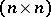-matrices oversuch that:is closed with respect to the Hermitian adjoint, which is defined by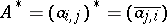for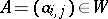;, whereis the unit matrix;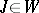, whereis the all-one matrix;is closed with respect to Schur–Hadamard multiplication, where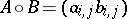for,,. Each coherent algebrahas a unique basis of zero-one matricessuch that:

1)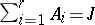;

2), where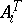is the matrix transposed to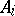;

3)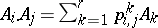. Property 1) implies that the basis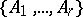consists of mutually orthogonal idempotents with respect to the Schur–Hadamard product. This basis is called the standard basis of. The non-negative integer structure constants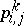are important numerical invariants of. The notation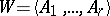indicates thatis a coherent algebra with standard basis.

Let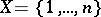and denote bya binary relation over.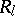is called the support of the zero-one matrix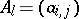(or, in other words,is the adjacency matrix of the graphwith vertex setand setof directed edges). The system of relations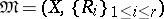obtained in this way from a coherent algebrais called a coherent configuration.

The structure constantsofare sometimes called the intersection numbers of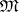. They have the following combinatorial interpretation: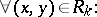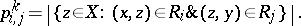A coherent configurationis called homogeneous if one of its basic relations, say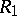, coincides with the diagonal relation. In terms of matrices, a coherent algebrais called a Bose–Mesner algebra (briefly BM-algebra) if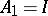. Note that according to E. Bannai and T. Ito [a1], a homogeneous coherent configuration is also called an association scheme (not necessarily commutative; cf. also Association scheme).

Letbe a coherent configuration. A subsetis called a fibre ofif(a1)

andis a minimal (with respect to inclusion) subset satisfying condition (a1). The coherent algebras with one fibre are exactly the BM-algebras. Coherent algebras with few fibres may be used for a unified presentation and investigation of various combinatorial objects, see, for example, [a3], [a7], [a9].

An important class of coherent algebras consists of the centralizer algebras of permutation groups (not necessarily transitive) [a2], [a10] (cf. also Permutation group; Centralizer). This leads to many important applications of coherent algebras.

It was Higman [a5], [a8] who developed the foundations of the representation theory of coherent algebras as a generalization of the representation theory of finite permutation groups (cf. also Finite group, representation of a).

How to Cite This Entry:
Coherent algebra. Encyclopedia of Mathematics. URL: http://encyclopediaofmath.org/index.php?title=Coherent_algebra&oldid=17154
This article was adapted from an original article by Mikhail Klin (originator), which appeared in Encyclopedia of Mathematics - ISBN 1402006098. See original article